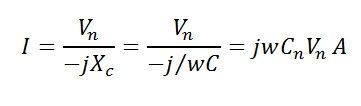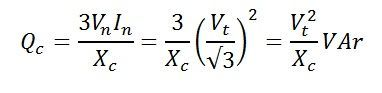# Charging Current in Transmission Line

In a transmission line, air acts as a dielectric medium between the conductors. When the voltage is applied across the sending end of the transmission line, current starts flowing between the conductors (due to imperfections of the dielectric medium). This current is called the charging current in the transmission line.In other words, we can say, the current associated with the capacitance of a line is known as the charging current.The strength of the charging current depends on the voltage, frequency, and capacitance of the line. It is given by the equations shown below.

For a single-phase line, the charging currentWhere, C= line-to-line in farads
Xc= capacitive reactance in ohms
V= line voltage in voltsAlso, reactive volt-ampere generated by the line = charging volt-amperes of the linesFor a three phase line, the charging current phasewhere Vn =voltage to neutral in volts = phase voltages in volts
Cn = capacitance to neutral in faradsReactive volt-ampere generated by the line = charging volt-amperes of the lineswhere Vt = line-to-line voltage in volts.

### Significance of charging current

1. It reduces the load current, due to which line losses decreases, and hence the efficiency of the line is increased.
2. It improves the power factor of the transmission line.
3. Charging current improves the load capacity of the line.
4. It improves the voltage regulation of the line because the voltage drop is quite small.

### 10 thoughts on “Charging Current in Transmission Line”

1. in my transmission line trainer, when i energize the line at no load, the voltage at the receiving end is higher than the sending end. is this caused by the charging current? how do you explain this? is this true in the real transmission line?
Thank you.

1. there is the ferranti effect in your transmission line. because in ferranti effect there are high voltages at receiving end. By using shunt reactors at receiving end of your line you can find your desired voltage.

2. The effect of line capacitance is to produce charging Current, which has a max value at which end of line???